# Vtu2Grid

## Introduction

This utility rasterises an existing 3D mesh at a given resolution. The result is a (quasi-)structured grid (consisting of hexahedral elements) with the same extent as the input mesh. Cell properties are mapped onto the grid (sampled at centre-points of each cube), while node properties are ignored. For large raster sizes, an undersampling of the original mesh is possible.

The tool is part of the official OpenGeoSys git repository.

## Usage

   Vtu2Grid  -i <input.vtu> -o <output.vtu> -x <floating point number>
[-y <floating point number>] [-z <floating point number>]

Where:

-i <input.vtu>,  --input <input.vtu>
(required)  the 3D input mesh (*.vtu, *.msh)

-o <output.vtu>,  --output <output.vtu>
(required)  the output grid (*.vtu)

-x <floating point number>,  --cellsize-x <floating point number>
(required)  edge length of cubes in x-direction (longitude) or all
directions, if y and z are not set

-y <floating point number>,  --cellsize-y <floating point number>
edge length of cubes in y-direction (latitude)

-z <floating point number>,  --cellsize-z <floating point number>
edge length of cubes in z-direction (depth)

The x/y/z-parameters determine the raster size. If only x is given, all raster cells will be (equilateral) cubes. If all three parameters are specified, the programme will create rectangular cuboid cells.

## Simple example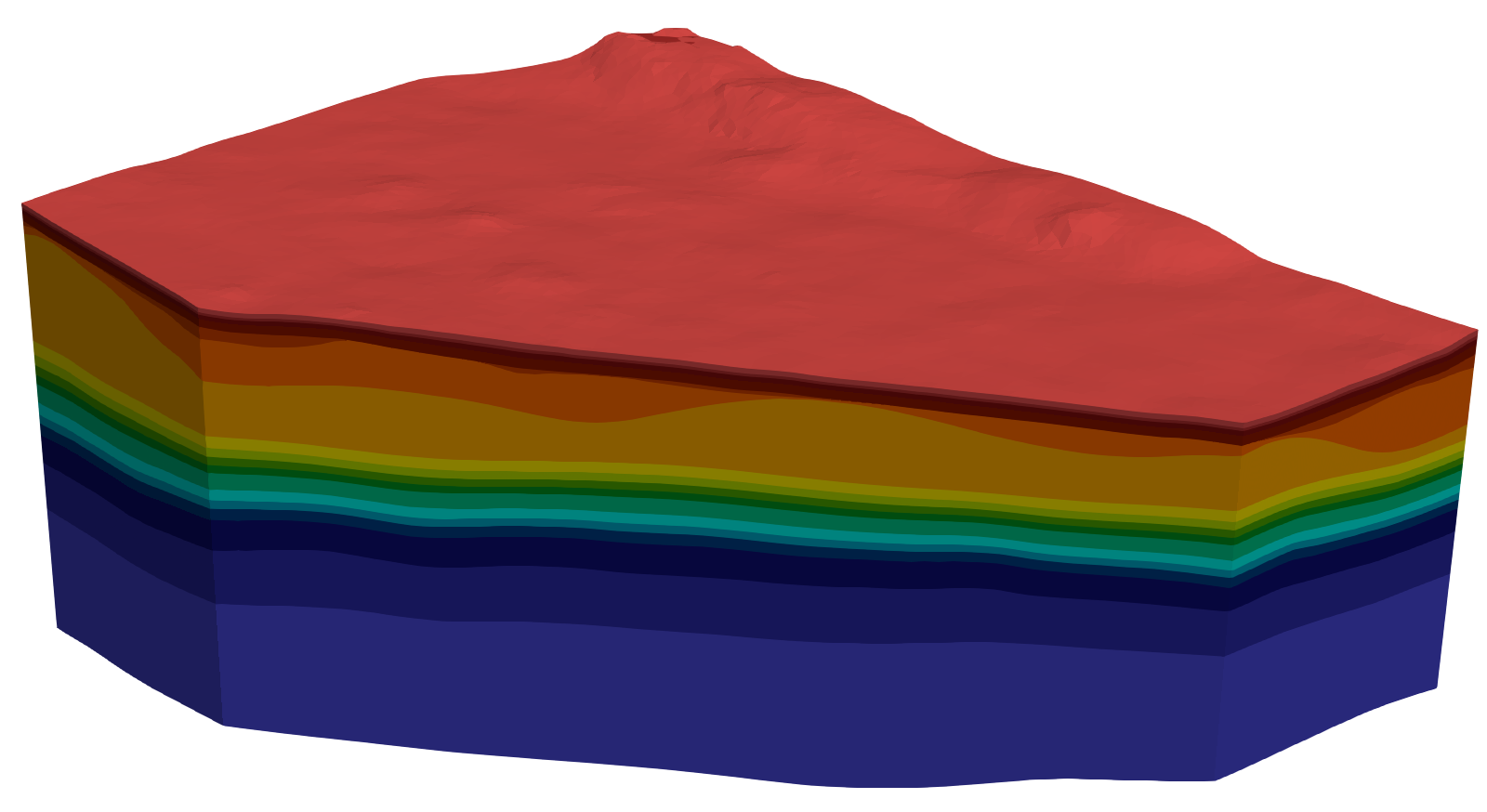Original, unstructured grid consisting of 217,128 prism-elements. The subsurface represented by this mesh consists of a number of layers of very different thickness. The very thin layers at the top (reddish tones) and in the middle (green and cyan tones) are particularly difficult to handle during numerical simulation.

Command:

Vtu2Grid -i input.vtu -o output.vtu -x 200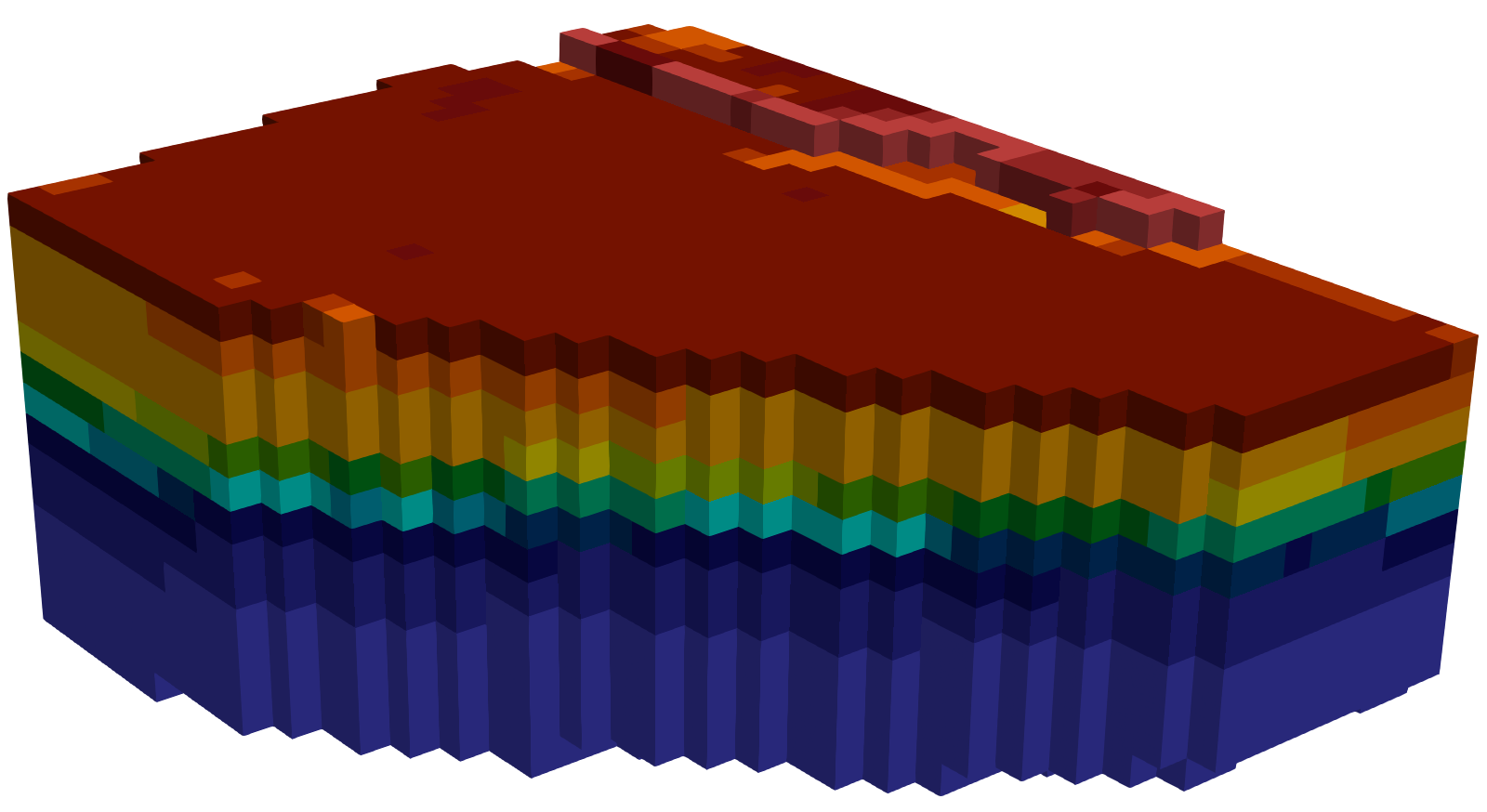Rasterised grid consisting of 9,240 cubes (equilateral hexahedral elements with an edge length of 200m). The result is severely undersampled and a continuous layer structure is no longer visible.

Command:

Vtu2Grid -i input.vtu -o output.vtu -x 100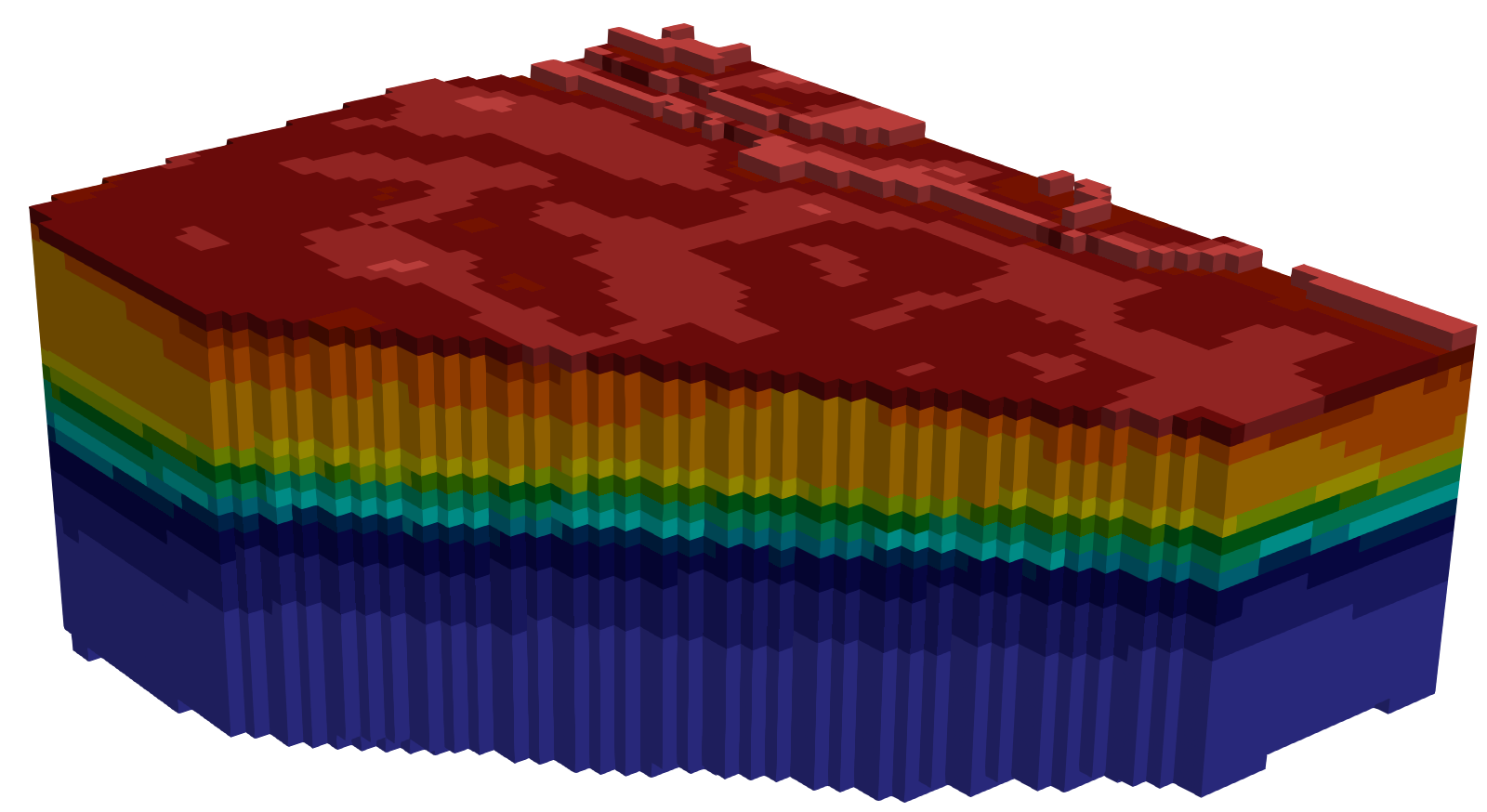Rasterised grid consisting of 74,048 equilateral hexahedral elements with an edge length of 100m. The result is still undersampled but layers become already visible.

Command:

Vtu2Grid -i input.vtu -o output.vtu -x 50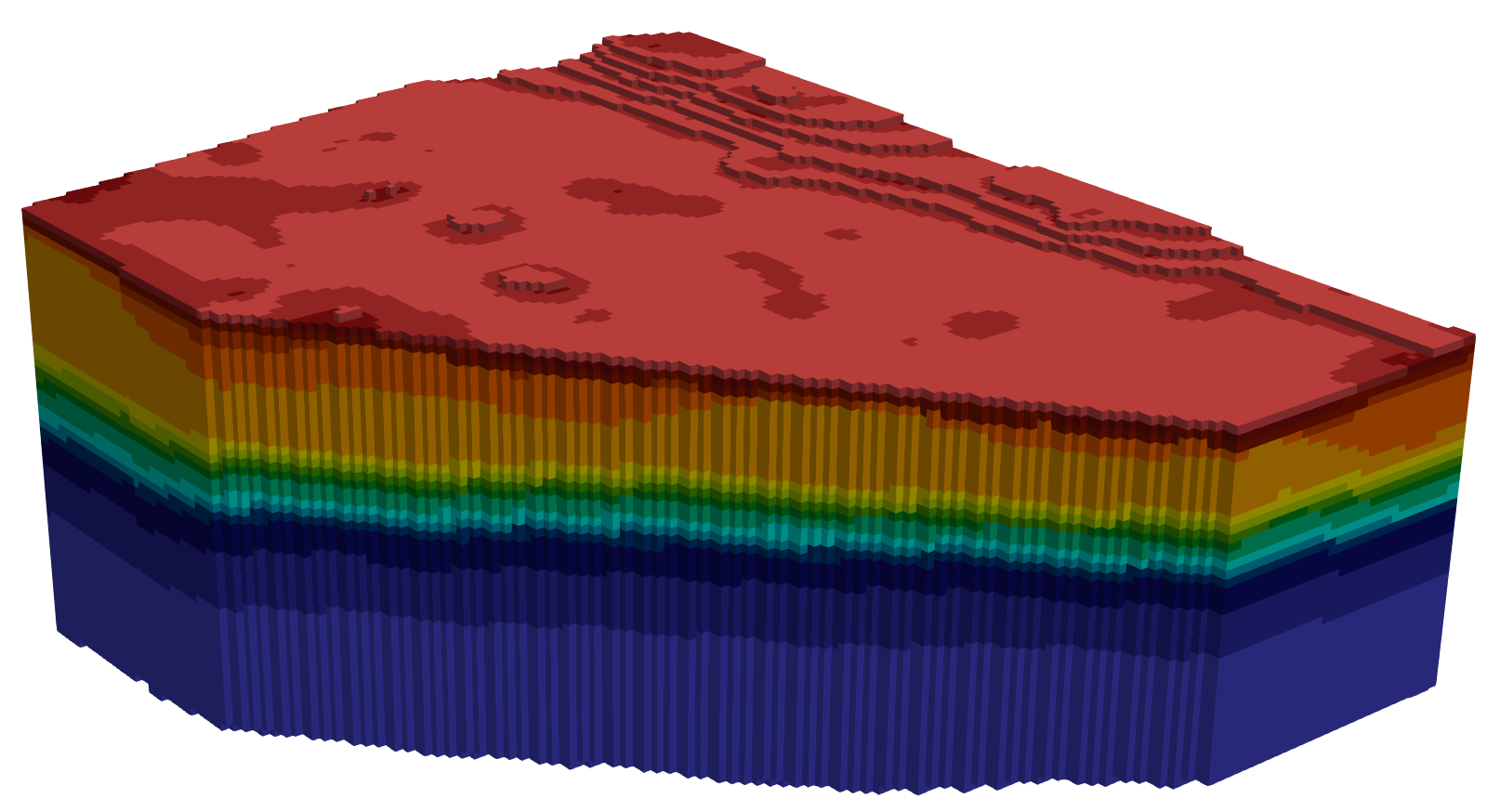Rasterised grid consisting of 591,757 equilateral hexahedral elements with an edge length of 50m. There’s still undersampling in regions containing thin layers but the overall structure is reasonably well represented.

Command:

Vtu2Grid -i input.vtu -o output.vtu -x 50 -y 50 -z 10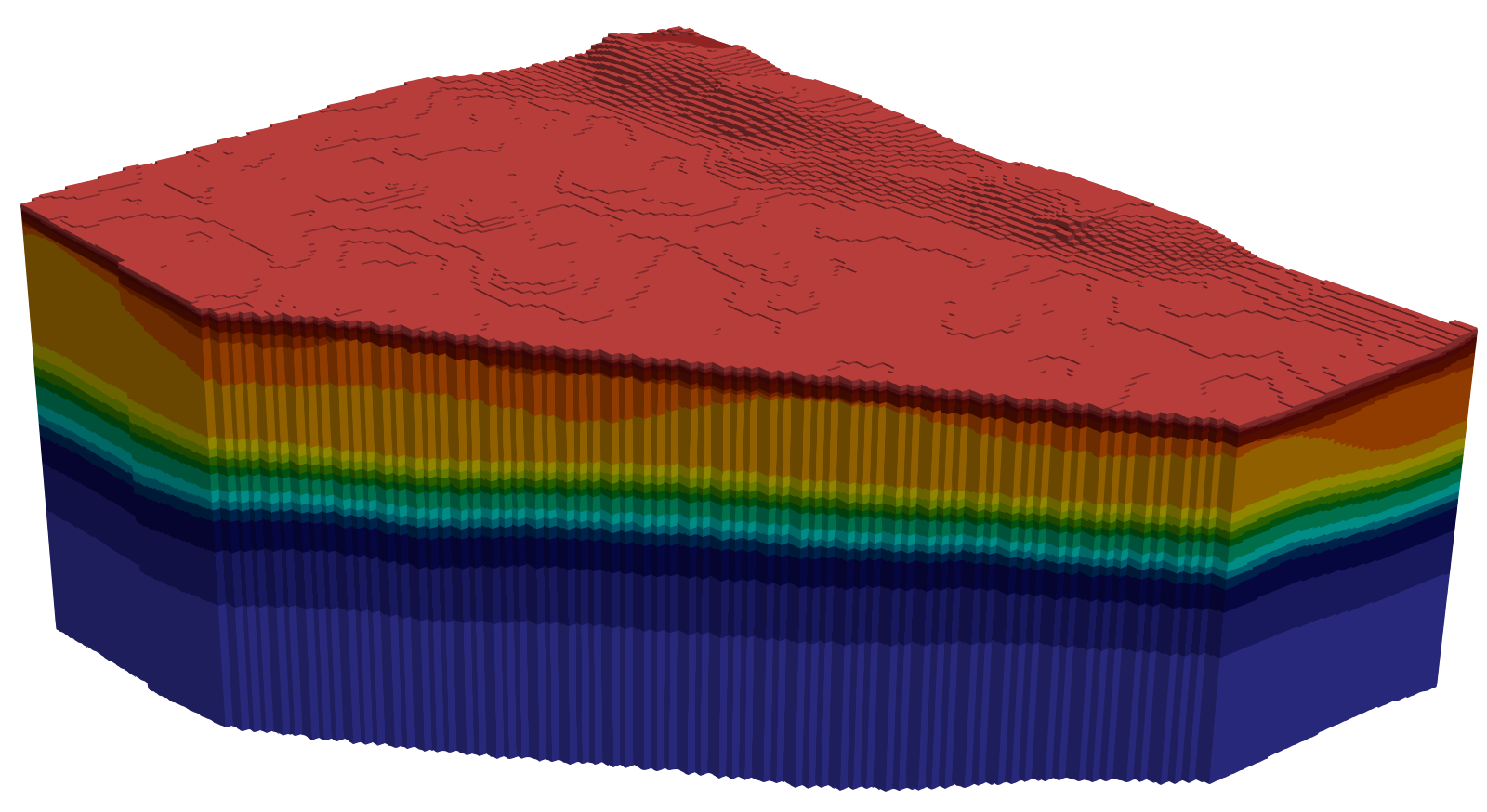Rasterised grid consisting of 2,959,656 cuboid hexahedral elements with an edge length of 50m x 50m x 10m. The structure of the original mesh is very well represented while the number of elements has increased by an order of magnitude.

## Application

This utility can be used to convert complex unstructured 3D meshes with a numerically challenging geometry into simple, rasterised meshes. This will (significantly) increase the number of elements but prevents numerical issues during a subsequent simulation, thus exchanging a challenging model setup with a longer runtime. As such, the output mesh allows to quickly set-up a working model of region of interest to get first results that allow for informed decisions for a more realistic model using the unstructured input mesh.

Previous

This article was written by Karsten Rink. If you are missing something or you find an error please let us know. Generated with Hugo 0.60.1. Last revision: December 5, 2019